## Weighted Regression — Is it cheating?

Linear and non-linear regression are key tools that scientist apply regularly to model their data. This ranges from setting up a linear calibration line up to modelling biochemical processes like enzyme-substrate or drug-taget binding. If the measurement, or at least the analysis, is confined to a narrow range of responsens, homogeneity of variance is often a valid assumption. If, for instance, a linear calibration data set from a spectrophotometer ranges from 0.5 to 1.0 OD (OD = optical density), this assumption may (approximately) hold. If, however, the response range is much broader, one may realize that the scatter among replicates is much higher at higher OD values than at lower ones. In these cases it is worth thinking about using weighted regression instead of “conventional” unweighted regression.

Homoegeneity of variance means that, given the correct fit model, the variance of the errors or residualsis constant. This is commonly known as homoscedasticity. The residualscorrespond to the difference of the actual response values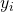and the predicted response values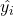of the fit model, i.e.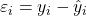. The residuals correspond the blue vertical lines in the following graph. They can be positive or negative.

A comparison between homoscedatic and heteroscedastic residuals is done in the following figure.

The homoscedastic residuals (left) uniformly scatter around zero while the magnitudes of the heteroscedastic residuals tend to increase with increasing predictor values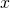.

In cases like this, it is quite common to apply an inverse variance weighting, where the variance of the replicates at each predictor is used as a weighting factor within the weighted least-squrare regression: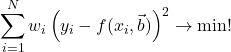Herein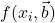denotes the fit model with predictorsand fit model parameter vector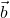. The expression above is the weighted sum-of-squared error being minimized within the weighted regression framework. For “conventional” regression all the‘s are 1. Sometimes the variances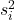is based on historical data, but sometimes, as said, they are estimated from the variances of the replicates at each predictor variables. However, one should be cautious with the latter approach as this requires quite a few replicates to get a trustworthy variance estimates right.
For dose-response curves another weighting scheme is quite common, i.e.. This means that the predicted data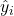itself is used to generate weights and thus account for increasing response-related variability. The problem here is that this always requires an iterative approach for the regression, even for a linear regression model (-> iteratively reweighted least-square, IRLS). Alternatively, one can try to use the averages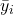of the response values here,. In practice, this requires multiple replicates per predictor but at the end it gives similar results as weighting with the predicted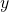-values and does not even require an iterative approach. It can simply be calculated by linear algebra:Where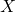denotes the Jacobian matrix,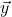the vector of responses and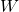denotes the square weight matrix with the weightson its diagonal. Againdenotes the vector of optimal fit model parameters. When the regression model is non-linear, the solution must, nonetheless, be obtained by an iterative approach.

In the following video I demonstrate how to perform an enzyme kinetic data analysis according to Lineweaver and Burk by applying a double-reciprocal transformation and subsequent weighted linear regression analysis.

How to check that my weighting is appropriate?

An easy way to check that the weighting is appropriate, is to plot the weighted residuals either against the predictor valuesor the predicted-values. If the weighting is appropriate the weighted residuals will be homoscedastic and equally scattered around the zero-line (see figure B below).

In this example the difference between the model lines of the weighted and unweighted regression is negligible. And in fact, this is often the case. Using the wrong weighting often doesn’t influence the fit model parameters so much, but it noticeybly affects their standard errors.

So, the standard errors are remarkably lower for the weighted linear regression compared to the unweighted case as we used the correct weighting scheme here.

Robust regression as weighted regression

One can choose the weightsin a way that data points that are far from the regression line do not influence the prediction so much. Again, this requires an iterative reweighting during the fitting. Say you’ve performed a conventional linear regression with all. Then you inspect the residuals and see that there are few data points that are far away from the fit line while most of the others are quite close to it. You might want to give the “outliers” less influence on the prediction and penalize them by assigning them lower weights: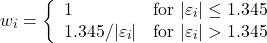So the idea here, and this is based on an idea of Peter Huber, is to not weight data points whose residuals are within the interval [-1.345, 1.345] and to assign lower weights to data points whose residuals are smaller than -1.345 or bigger than 1.345. These weights are sometimes referred to as Huber weights and are commonly used weights for robust regression. The Huber approach boils down to unweighted regression if there ar no outlying data points. There are others robust approaches, but these shall not be discussed within the scope of this blog post.

Is weighting cheating then?

The key point I want to make here is that without the residuals being homoscedastic, one of the basic assumptions of regression analysis is violated and the results derived from regression analysis might be improper and inaccruate. Weighting can help making heteroscedastic residuals become homoscedastic (i.e. weighted residuals).

What do you think is more cheating: Fitting a non-weighted model to “heteroscedastic data” or applying an appropriate weighting and then perform a weighted regression?

﻿

### Dr. Mario Schneider

Mario is an analytical chemist with a strong focus on chemometrics and scientific data analysis. He holds a PhD in biophysics and is the author of a book on data analysis for scientists. In his spare time he is a MATLAB, R, Excel and Python enthusiast and experiments with machine learning algorithms.